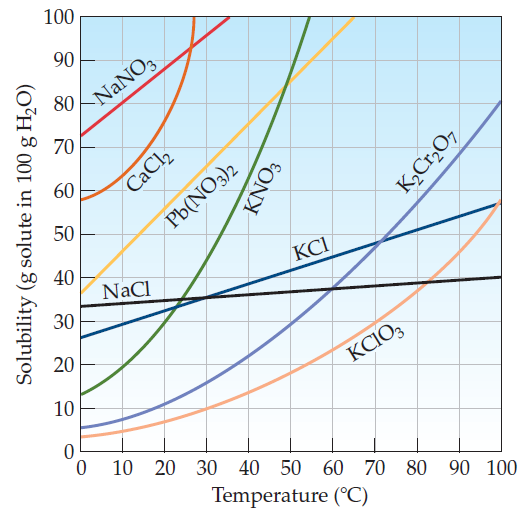×
Get Full Access to Introductory Chemistry - 5 Edition - Chapter 13 - Problem 33p
Get Full Access to Introductory Chemistry - 5 Edition - Chapter 13 - Problem 33p

×

# A KNO3 solution containing 45 g of KNO3 per 100 g of water is cooled from 40 °C to 0 °CISBN: 9780321910295 34

## Solution for problem 33P Chapter 13

Introductory Chemistry | 5th Edition

• Textbook Solutions
• 2901 Step-by-step solutions solved by professors and subject experts
• Get 24/7 help from StudySoup virtual teaching assistantsIntroductory Chemistry | 5th Edition

4 5 1 419 Reviews
30
2
Problem 33P

A KNO3 solution containing $$45 g$$ of KNO3 per $$100 g$$ of water is cooled from $$40^{\circ} \mathrm{C}$$ to $$0^{\circ} \mathrm{C}$$. What happens during cooling? (See Figure $$13.4$$.)

Figure $$13.4$$ Solubility of some ionic solids as a function of temperatureEquation Transcription:KNO340°C

0°CText Transcription:

45 g

KNO_3

100 g

40 degree C

0 degree C

13.4

Step-by-Step Solution:

Step 1 of 2

The solubility of a solid in a solution is dependent on temperature. Thus the solubility of most solids in water increases with increasing temperature.

From the figure 1., it is observed the solubility ofatis 62 g per 100 g of water whereas the solubility ofatis 12 g per 100 g of water. Thus, asolution containing 45 g of KCl per 100 g of water is cooled atforms unsaturated solution.

Step 2 of 2

##### ISBN: 9780321910295

Introductory Chemistry was written by and is associated to the ISBN: 9780321910295. The answer to “?A KNO3 solution containing $$45 g$$ of KNO3 per $$100 g$$ of water is cooled from $$40^{\circ} \mathrm{C}$$ to $$0^{\circ} \mathrm{C}$$. What happens during cooling? (See Figure $$13.4$$.)Figure $$13.4$$ Solubility of some ionic solids as a function of temperature Equation Transcription:KNO340°C0°CText Transcription:45 gKNO_3100 g40 degree C0 degree C13.4” is broken down into a number of easy to follow steps, and 48 words. The full step-by-step solution to problem: 33P from chapter: 13 was answered by , our top Chemistry solution expert on 05/06/17, 06:45PM. Since the solution to 33P from 13 chapter was answered, more than 4526 students have viewed the full step-by-step answer. This full solution covers the following key subjects: kno, figure, containing, during, cooled. This expansive textbook survival guide covers 19 chapters, and 2046 solutions. This textbook survival guide was created for the textbook: Introductory Chemistry, edition: 5.

## Discover and learn what students are asking

Unlock Textbook Solution